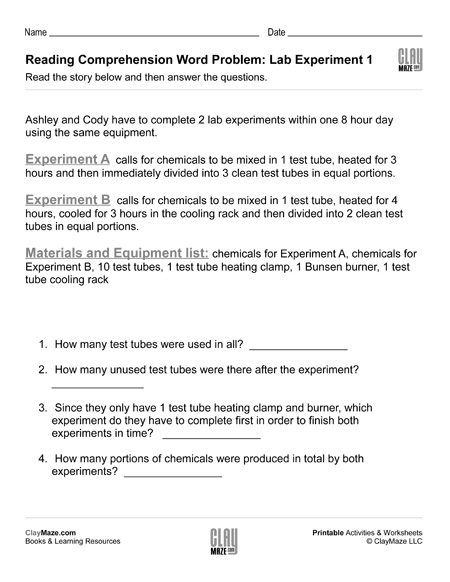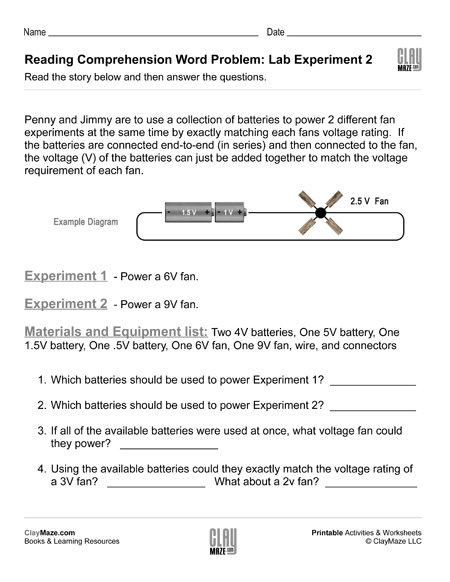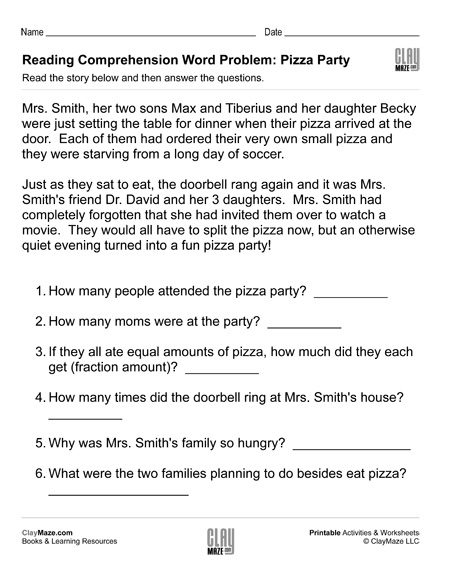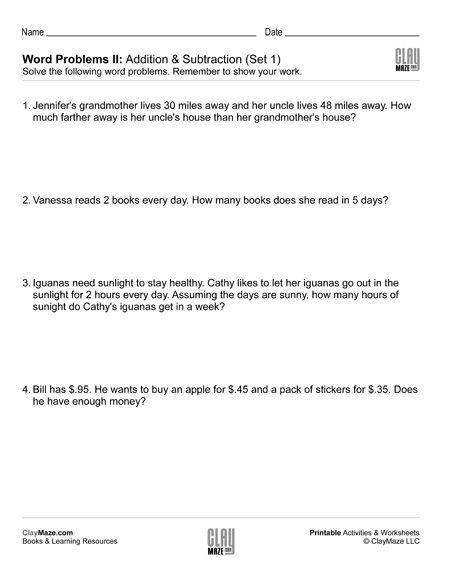## Topic: Word Problems

Free Printable Worksheets with Word Problems

## Reading Comprehension Word Problem: Lab Experiment 1This is the first of a 3 part set of worksheets which combines reading comprehension, word problems and a science lab experiment environment.  Science experiments are a fun bridge between the phys...

## Reading Comprehension Word Problem: Lab Experiment 2This worksheet is the second part of a three part reading comprehension word problem series.  The set is designed as a STEM resource with an introduction to scientific experiment as well as...

## Reading Comprehension Word Problem: Pizza PartyAs our subject matter becomes more and more technical and complex, it becomes increasingly important for children to learn to read carefully and re-read a passage in order to fully grasp the...

## Reading Comprehension Word Problem: Lab Experiment 3This free children’s worksheet is the 3rd part of a 3 part series which combines scientific experiment with word problems.  Exercises like these are a great bridge between early learning...

## Word Problems I: Addition & SubtractionThese are sets of basic addition and subtraction word problems. The student should read through each problem thoroughly to answer the questions and be sure to show their work below each one. These ...

## Word Problems II: Addition & SubtractionThese sets of word problems are slightly more challenging than the Word Problems I sets. The student will need to use addition and subtraction to solve, but some problems use a little bit larger nu...

## Time Word Problems: Analog ClocksThese sets of worksheets are great for practice telling time with analog clocks and for understanding how to add and subtract with different time intervals. On these worksheets, the student is aske...